# Investigation of the magnetic and magnetodielectric properties of few complex spinels

N/A
N/A
Protected

Share "Investigation of the magnetic and magnetodielectric properties of few complex spinels"

Copied!
185
0
0

Full text

The investigation of the magnetic and magneto-dielectric properties of some complex spinels" is the result of my doctoral research work. A systematic comparison of the magnetic properties of Co2SnO4 samples sintered at two different temperatures, 1200ºC (S1200) and 1350ºC (S1350), is reported.

The inset (i) and (ii) of shows the peak variation clearly in logarithmic scale. sample as a function of the applied magnetic field H. The inset shows variation of the transition temperatures TP1 and TP2 as a function of Hdc2/3 for Co2Ti0.6Sn0.4O4. The scale on the right shows the variation of high-field (H~ 68.3 kOe) magnetization versus temperature measured under both (a).

List of Symbols

## Introduction to Spinels and their Complex Magnetic Structure

In general, spinel can be classified into two categories (i) Normal spinels: (A2+)tetra[B3+2]octX2−4, in this configuration all the 'A' cations occupy the tetrahedral voids and all the 'B' cations occupy the voids octahedral. . Example: MgAl2O4, CoCr2O4, Co3O4, Mn3O4, etc., (ii) Inverse spinels: (B3+)tetra[A2+B3+]octX2−4, in this configuration half of the B cations occupy the tetrahedral void and the rest of the 'B' cations together with 'A' sit in the octahedral voids.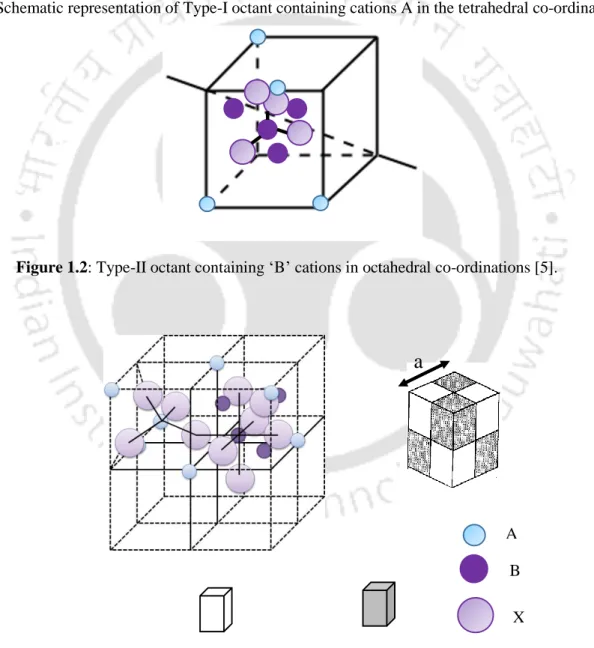Figure 1.1: Schematic representation of Type-I octant containing cations A in the tetrahedral co-ordinations 

## SOFC Li-ion

### Nature of Magnetic Ordering in Co 2 SnO 4

The asterisk shown in Figure 3.1 corresponds to the peak due to unreacted nonmagnetic SnO2. Such a shift of the peak position towards higher temperatures as in χ'(T) and χ''(T) clearly implies the existence of glassy behavior in the investigated systems.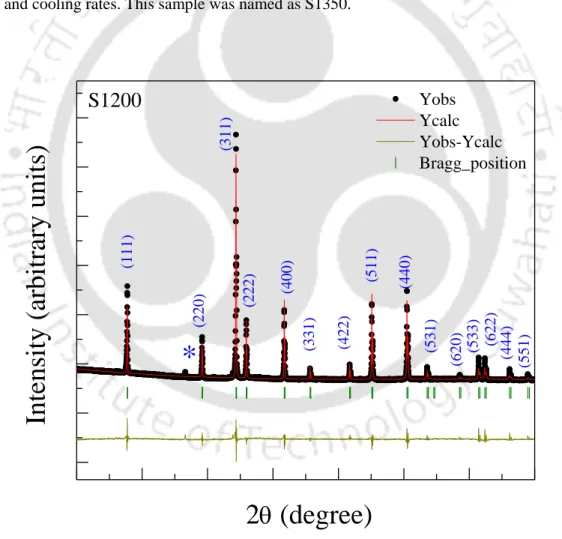Figure 3.1: The X-ray diffraction pattern (dotted symbols) together with the Rietveld refined data (red line) of Co 2 SnO 4 bulk samples sintered at 1200 º C (S1200) in air

The insets (a)–(c) show the zoom view of the same curves at different temperature regimes to better represent the transition. Such a type of semi-spin glass state was first predicted by Villian in magnetically dilute systems and later established theoretically by Gabay and Thoulouse [40, 122]. Such behavior justifies the ferrimagnetic character of Co2SnO4, where the direct coupling occurs between the order parameter and the applied field.

The solid lines show the linear fit to the data (often called the de Almeida-Thouless AT line) while the dotted line for TSG is for visual aid.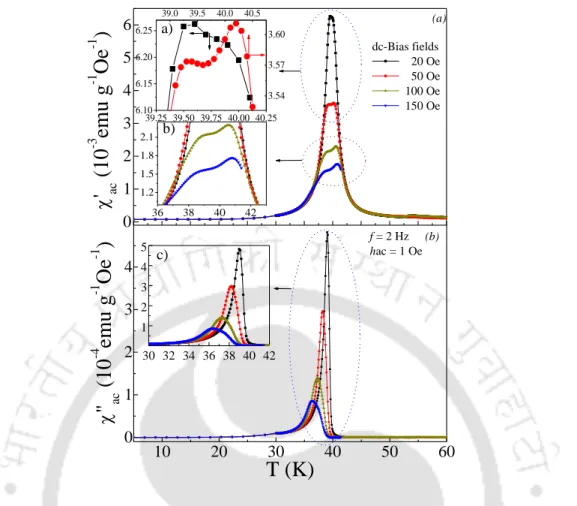Figure 3.12: Temperature dependence of ac-magnetic susceptibilities: (a) real part () and (b) imaginary part () component of S1200 recorded at various dc-bias fields H dc = 20, 50, 100 and 150 Oe with constant frequency f = 2 Hz and amplitude

## T SGfrom d('T)/dT

Such 𝐻𝑑𝑐2/3 variation of the peak is characteristic of the de Almeida-Thouless line (popularly known as AT line) for a spin-glass state and such variation is often used as one of the tests for the presence of short-range spin-glass-like ordering in a variety of disordered systems. Application of the external DC magnetic field reduces this random tilt and shifts TSG to a lower temperature value. The magnitudes of the loop asymmetry parameter HEB for the ZFC samples were also determined from the measured hysteresis loops (Figure 3.21).

Using the adjusted parameters of the Néel's expression we evaluated the molecular field constants (NAA, NBB and NAB) between nearest neighbor and nearest neighbour, and the corresponding exchange constants (JAA, JBB and JAB) for both S1200 and S1350 systems .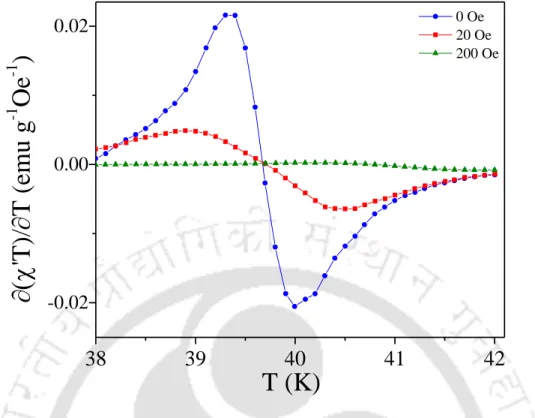Figure 3.15: Temperature variation of ( ac T)/T plots at different bias fields 0 Oe ≤ H dc ≤ 200 Oe for S1350 sample

## T (K)HEB (Oe)

It can be clearly observed from the table that the molecular field constants follow the trend NAB > NAA > NBB. From the above analysis, we can conclude that the difference in µeff for Co2+ at A and B sites along with the antiferromagnetic coupling constants is responsible for the ferrimagnetism in Co2SnO4. The solid lines fit Neel's expression for ferrimagnets, as discussed in this chapter.

In 1956, Anderson predicted that in normal spinels such as ZnFe2O4, in which all the magnetic Fe3+ ions occupy B-sites, and geometric frustrations would result in a lack of long-range magnetic order .

## T (K)1/ (emu-1mol-Oe)

There is another rapid decay in CP/T with decreasing T starting near 15 K, indicating further changes in the magnetic ordering of the system. Further support for these results comes from the temperature dependence of the magnetic hysteresis loop parameters discussed in section (b). A quantitative explanation of the temperature dependence of HC and MR in Co2SnO4 shown in Figures 3.19 and 3.20 is not yet possible.

In magnetic properties, the biggest noticeable difference between the two samples is in the magnitudes of coercivity HC.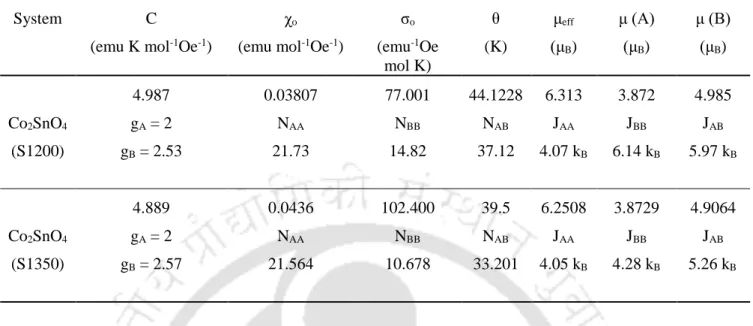Table 3.2: The list of various parameters obtained from the Néel fits of  -1 versus T curve recorded under zero- zero-field condition

## Negative Magnetization and Compensation Effect in Co 2 TiO 4

We also investigated the similarities and differences in the magnetic properties of the isostructural compounds Co2TiO4 and Co2SnO4. Such variation in the lattice parameters is generally associated with the different ionic radii of the constituent. In the case of Co2TiO4, however, the maximum intensity peak for Ti-2p3/2 appears at 457.65 eV (figure 4.2d), while the second maximum intensity.

The solid lines are best fits to the Néel expression for ferrimagnets discussed in Section 4.3.3.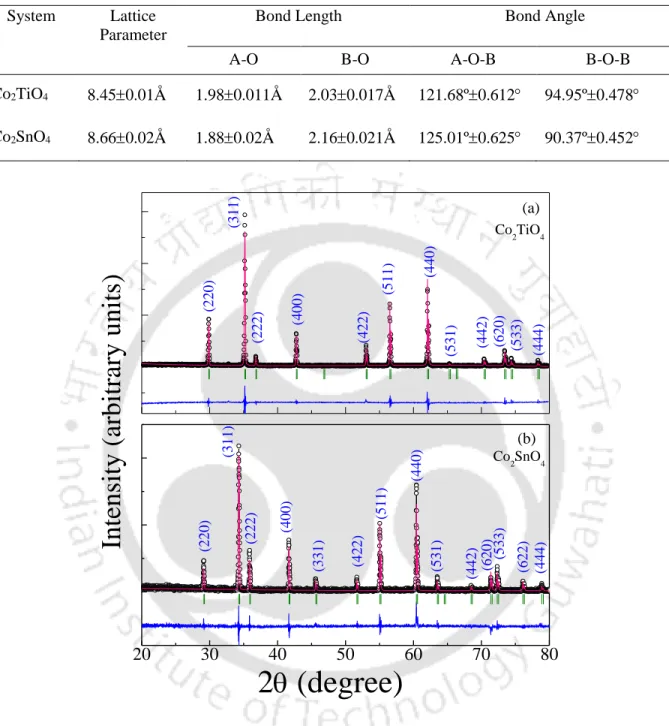Table 4.1: The list of lattice parameters (a = b = c), bond lengths and bond angles in Co 2 SnO 4 and Co 2 TiO 4

The inset of figure 4.11 shows asymmetry in the MH loops measured at 5 K under ZFC and FC (± 20 kOe) protocol. In the Stoner-Wohlfarth (SW) model of coercivity in single-domain particle, HC = KA/MS, where KA is the magneto-crystalline anisotropy constant and MS is the saturation magnetization [124, 163]. A significant deviation from positive slope of the Arrott plots can be clearly seen in the region T ≤ TCOMP ≤ TN (figure 4.15).

Graphs of the temperature dependence of the specific heat CP(T) of Co2TiO4 measured at Hdc = 0, 10 kOe and 50 kOe are shown in Figure 4.18.

## T (K)CPT-1 (J mol-1)

It is known that the total specific heat CP(T) consists mainly of two parts (i) lattice specific heat (CPlatt) (ii) magnetic contribution (CPmag). Since the electronic contribution is important only at very low temperatures, the phonon contribution is deduced from the total specific heat. The extrapolation data for both systems are shown in figure 4.21 together with the individual contribution of the phonon and magnetic components obtained from the above relation [172, 173].

Moreover, temperature variation of the differential magnetic entropy ∂SM/∂T (= CMP/T) shows a sharp peak above TCOMP of Co2TiO4 at high fields (≥ 50 kOe), typical of a first-order-like transition, but this field-dependent anomaly is not sharp at low fields (figure 4.22).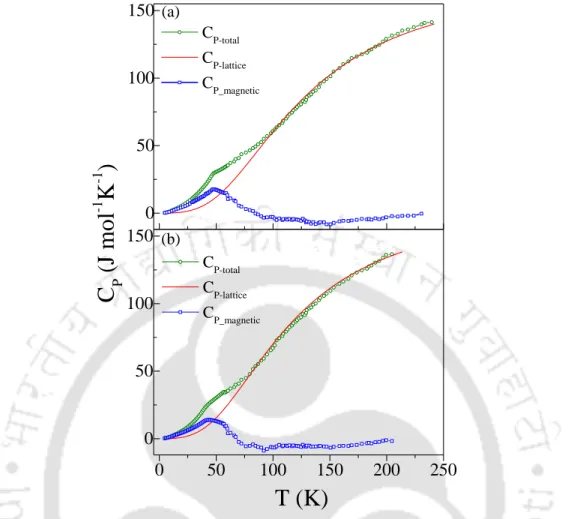Figure 4.21: Temperature dependence of experimentally obtained zero-field heat capacity data of (a) Co 2 TiO 4 and (b) Co 2 SnO 4 shown in green color open circles together with the red color solid line represents the specific heat calculated from nu

In the low temperature regime, the logarithmic plot of CP versus T shows a linear variation with slope ~ 1.91 (Figure 4.23). In these compounds, the long-range order is set by geometric frustration and the collective excitations of the spin-fluid; as a result, the magnetic heat capacity shows T2 dependence [175, 176]. Therefore, anomalous T2 dependence in heat capacity data in this study clearly suggests that the spin-liquid-like excitations exist in both Co2TiO4 and Co2SnO4 with non-Heisenberg magnetic interactions at low temperatures.

This chapter presents a systematic comparative analysis of the structural and magnetic properties of both Co2TiO4.

## T (K)CP ( J mol-1K-1)

This difference in the electronic structures of the B-site ions was used to explain the difference in the observed values ​​of μ(B) and the lack of reliable evidence for the presence of a spin-glass transition in Co2TiO4 in contrast to the observation in Co2SnO4; (iii). The large HC coercivity values ​​observed in Co2TiO4 in the uncompensated state are most likely due to spin clusters. v) The low-temperature complex magnetic ordering of Co2TiO4 and Co2SnO4 was studied using temperature dependence magnetization (M), Arrott analysis and specific heat (CP). In the same temperature regime (5 K ≤ T ≤ 33 K), CP followed a T2 dependence instead of a linear variation in both systems, indicating a dynamic spin fluctuation at low temperatures.

The random distribution of cations at the A and B sites and the different temperature dependence of the magnetic moments (μ[A](T) and μ[B](T)) were the likely source of such anomalous low-temperature magnetic ordering in Co2TiO4 and Co2SnO4.

## Tuning the Compensation Point: Role of Sn Substitution in Co 2 TiO 4

Such tilted behavior plays an important role in the magnetic ordering of the samples, which is discussed below. Because theoretically the temperature variation of ∂(χdcT)/∂T is analogous to the temperature variation of the heat capacity CP(T) in the systems exhibiting antiferromagnetic interactions [6, 18]. This exercise leads to the determination of the Néel temperature 'TN'= 46.10 K (referred to as TP1, shown in Figure 5.3) and the second transition 'TP2'.

The increase of the coercive field below the TF may be due to the growth of the spin-glass component.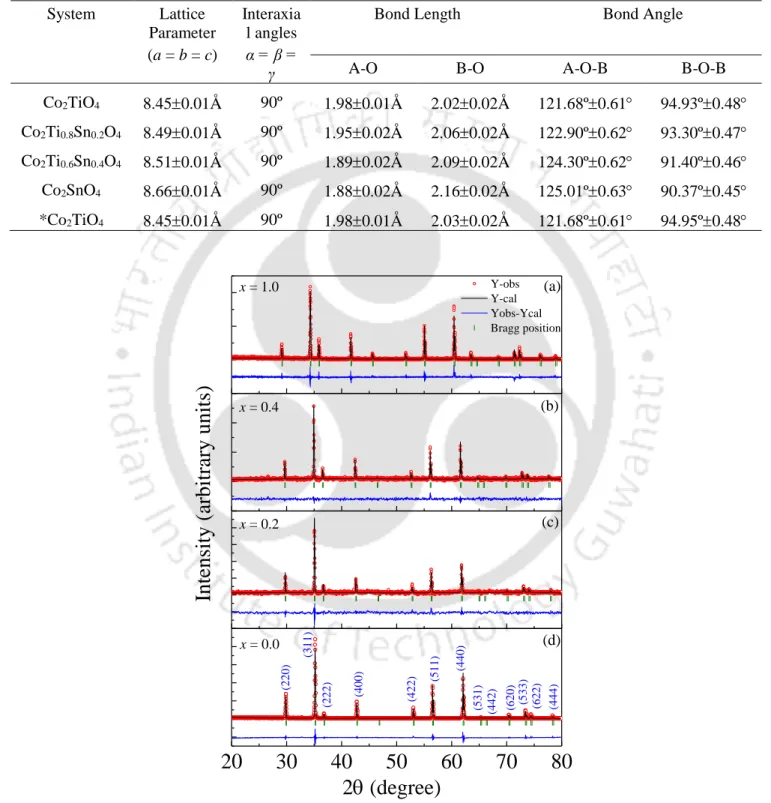Table 5.1: List of various crystal structure parameters (i) lattice parameter, (ii) bond Length and (iii) bond angles of various compositions of bulk samples Co 2 Ti 1-x Sn x O 4 (0 ≤ x ≤ 1)

## T(K)HEB (kOe)

Nevertheless, we believe that the reversal of the magnetic moments on either side of the TCOMP leads to the sign reversal of HEB. The scale on the right shows the variation of the high-field magnetization (H ~ 68.3 kOe) versus the temperature measured under both (a) ZFC and (b) FC conditions. The large magnitude of coercivity observed for tin substitute cobalt orthotitanate in the uncompensated state is most likely due to growth.

This effect is consistent with the change of the exchange integral (J) with respect to the composition Sn-(x), as shown in Figure 5.13b.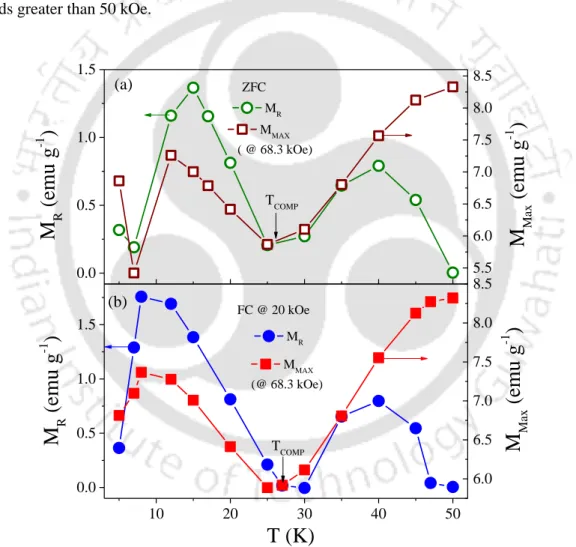Figure 5.11: Left-hand-side scale shows the remanence magnetization versus temperature (M R versus T) of Co 2 Ti 0.6 Sn 0.4 O 4 system measured under (a) zero-field-cooled (ZFC), and (b) field-cooled (FC) conditions

The unusual behavior of the intensity and the shape of the peak can be related to the distribution of cations in the solid solutions of the series (1-x) Co3O4 + x Co2TiO4. For a detailed understanding of the temperature-induced changes in the vibrational modes, we performed the low-temperature Raman measurements (down to 25 K) on both the systems Co3O4 and Co2TiO4. For a detailed understanding of the valence state of the cations, we performed the X-ray photoelectron spectroscopy (XPS) measurements for both the systems Co3O4 (x = 0) and Co2TiO4 (x = 1) in the form of sintered grains.

Large changes in the bond angles and bond lengths were noted for different compositions of the solid solutions (1-x) Co3O4 + x Co2TiO4.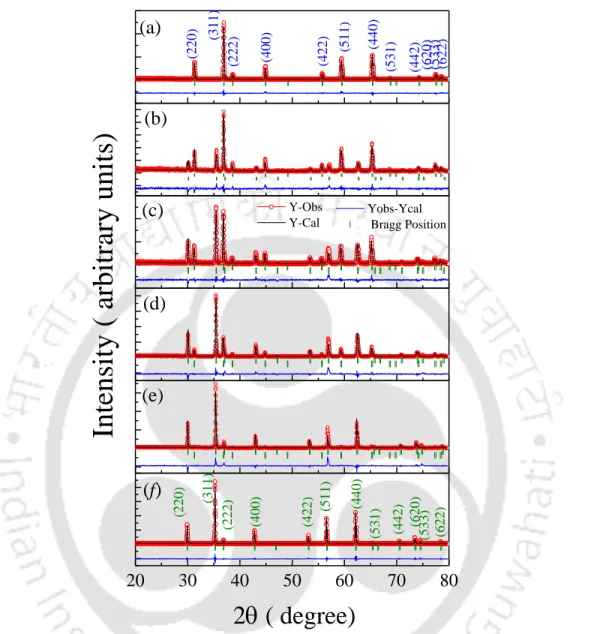Figure 6.1: The X-ray diffraction pattern together with the Rietveld refined data of composite system (1-x) Co 3 O 4

Since the average grain sizes of the Mn3O4 samples after quenching at 77 K are in the range 8 - 20 μm (figure 7.3), the irreversibilities cannot be due to blocking/spin-glass effects. The frequency (f) of the ac-magnetic field has a significant effect on all four transitions. The magnitude and trend of εr(T) obtained in polycrystalline samples are almost equal to the values ​​obtained with the out-of-plane dielectric constant (E║) versus temperature of single-crystal Mn3O4 .

This is the first report dealing with the temperature dependence of polarization measurements in Mn3O4 polycrystals.

## Conclusions and Scope for Future Research

All compositions exhibit two distinct bands at 576 cm-1 (B1) and 665 cm-1 (B2) in the Fourier transform infrared spectra recorded at 310 K which are associated with vibrational stretching of metal-oxygen bonds with length ~195.8 pm (B-O) and ~185.4 pm (A-O) respectively. We also observed a clear hysteresis of about 5.15 K in the zero field εr(T) along 34 K, which provides evidence for the first-order nature of this transition. In the current thesis we have explored only the structural, magnetic and electrical properties of spinel oxides Co2SnO4, Co2TiO4 and Mn3O4.

This study can be extended with various other doping elements, either non-magnetic or magnetic (e.g. Al, Mn, Ru, V and Cr on 'Ti' and 'Sn' sites) and investigate the differences occurring in the structural, optical and magnetic properties compared to the pristine compounds.

Bibliography

Figure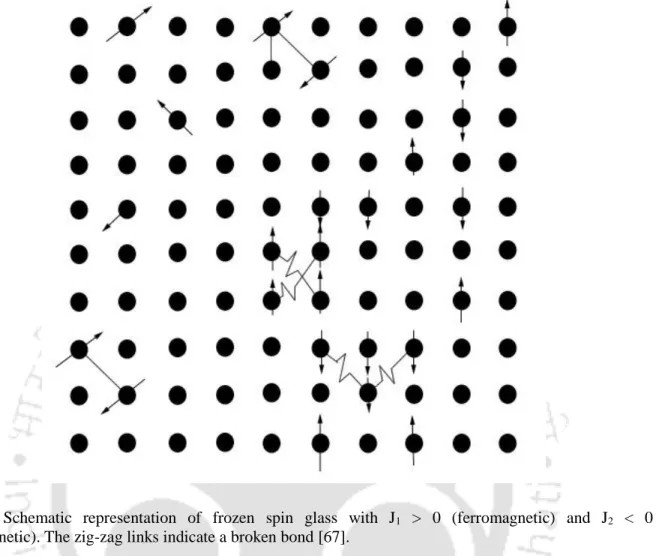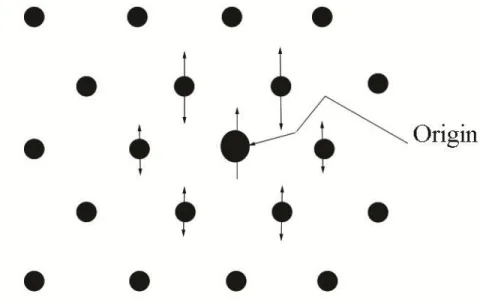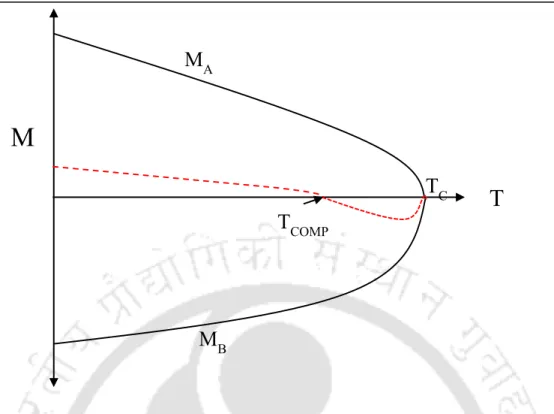+7

References

Related documents

[r]

i After successful completion of the course work, a candidate shall submit a synopsis of the proposed research work in triplicate to the Research Advisory Committee concerned through

Migration of Formerly Deported Peoples 1989-1999 Nation Deported Number Net When From To of people Migration Crimean Tatars 1942 Crimea Uzbekistan Data unavailable -105234 Volga

From Figure 2 left panel, we infer that the diurnal variation of the NS magnetic component, which shows two maxima, is possibly associated with the thunderstorm activity over Asia and

The concentration range of component proteins was chosen preferably from the linear region of area under the extinction curve Figure 2.8 Ratio of area under the UV-visible spectrum of

Residual effect on yield and yield attributes of toria Data in Table 3i and 3ii shows the residual effect of the main plot and sub-plot factor on the toria crop which shows that

Moholkar Thesis Submitted to the Department/ Center : Chemical Engineering Date of completion of Thesis Viva-Voce Exam : 11.07.2017 Key words for description of Thesis Work :

Chapter 1: Introduction demonstrated that the enhancement of LIBS signal of Cu plasma with magnetic field occured at 30 mJ.54 The behavior of LPP of different materials in the

Chapter 4 demonstrates the fabrication of a high temperature sustainable single component WLE nanocomposite, with properties close to day bright light in terms of the chromaticity,